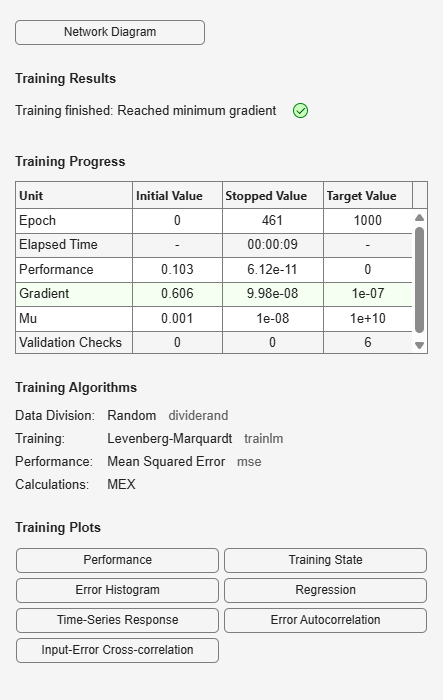Documentation

# layrecnet

Layer recurrent neural network

## Syntax

```layrecnet(layerDelays,hiddenSizes,trainFcn) ```

## Description

Layer recurrent neural networks are similar to feedforward networks, except that each layer has a recurrent connection with a tap delay associated with it. This allows the network to have an infinite dynamic response to time series input data. This network is similar to the time delay (`timedelaynet`) and distributed delay (`distdelaynet`) neural networks, which have finite input responses.

`layrecnet(layerDelays,hiddenSizes,trainFcn)` takes these arguments,

 `layerDelays` Row vector of increasing 0 or positive delays (default = 1:2) `hiddenSizes` Row vector of one or more hidden layer sizes (default = 10) `trainFcn` Training function (default = `'trainlm'`)

and returns a layer recurrent neural network.

## Examples

### Recurrent Neural Network

Use a layer recurrent neural network to solve a simple time series problem.

```[X,T] = simpleseries_dataset; net = layrecnet(1:2,10); [Xs,Xi,Ai,Ts] = preparets(net,X,T); net = train(net,Xs,Ts,Xi,Ai); view(net) Y = net(Xs,Xi,Ai); perf = perform(net,Y,Ts) ```
```perf = 6.1239e-11 ```